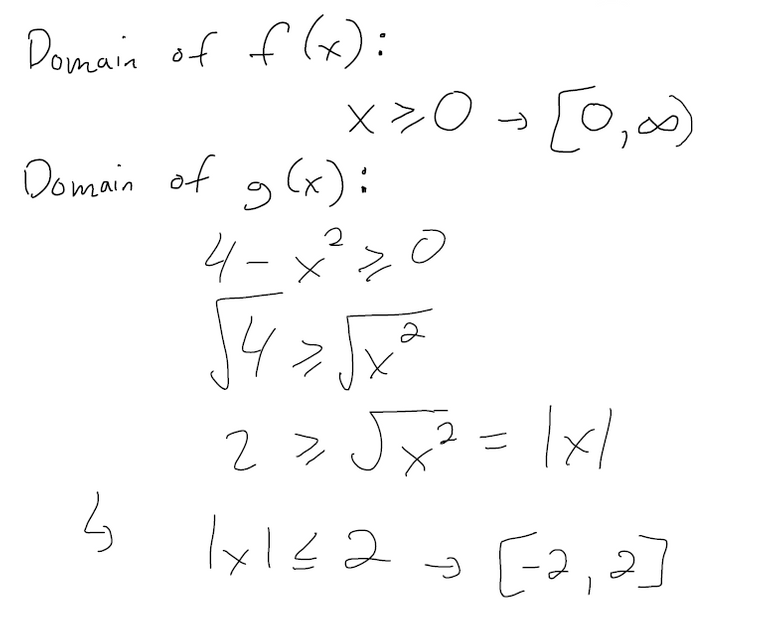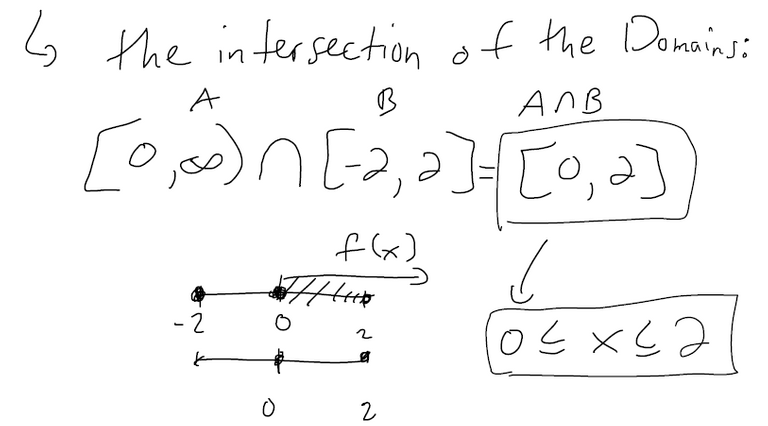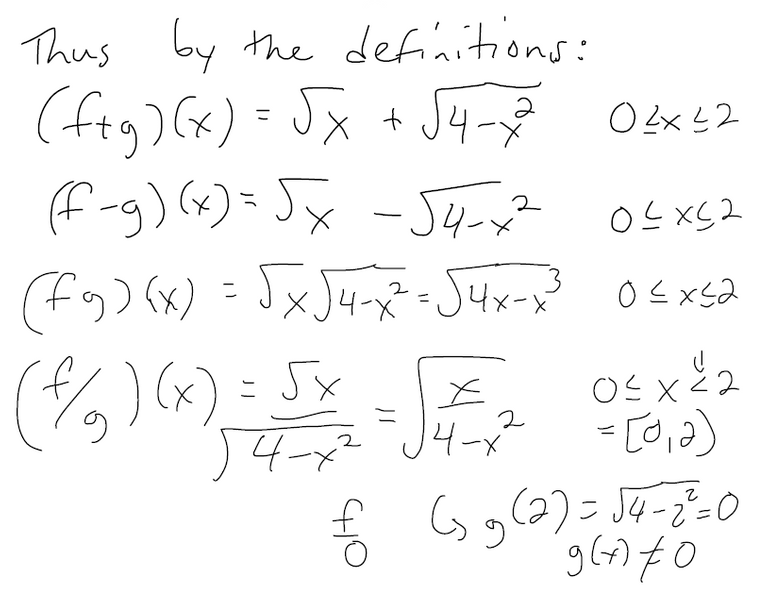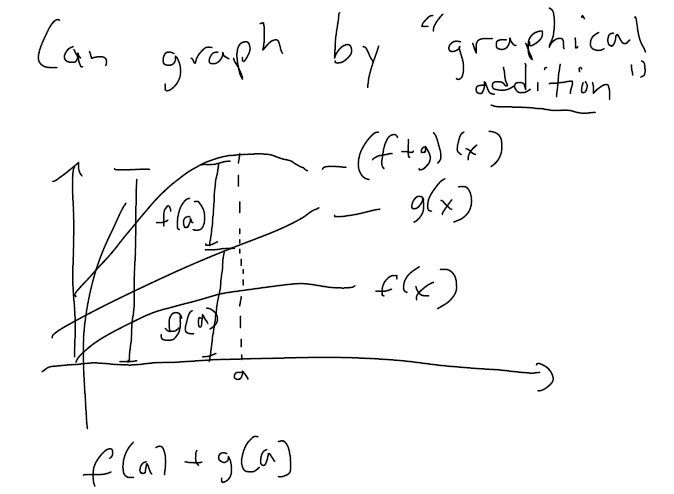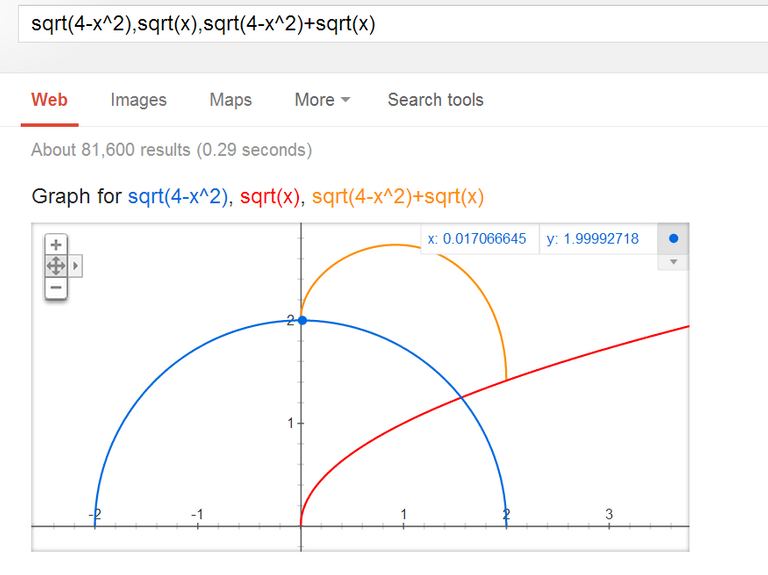# Combination of Functions: (f+g)(x), (f-g)(x), (fg)(x), (f/g)(x)

in MES Science3 months ago (edited)

In this video I go over the definitions and notations involved in combining two different functions. What I mean by combining functions, I mean adding, subtracting, multiplying and dividing two functions.

Watch video on:

# View Video Notes Below!

View these notes as an article: https://peakd.com/@mes
Subscribe via email: http://mes.fm/subscribe
Donate! :) https://mes.fm/donate

Reuse of my videos:

• Feel free to make use of / re-upload / monetize my videos as long as you provide a link to the original video.

Fight back against censorship:

• Bookmark sites/channels/accounts and check periodically
• Remember to always archive website pages in case they get deleted/changed.

Recommended Books:

Subscribe to MES Truth: https://mes.fm/truth

Join my forums!

Follow along my epic video series:

NOTE #1: If you don't have time to watch this whole video:

Browser extension recommendations: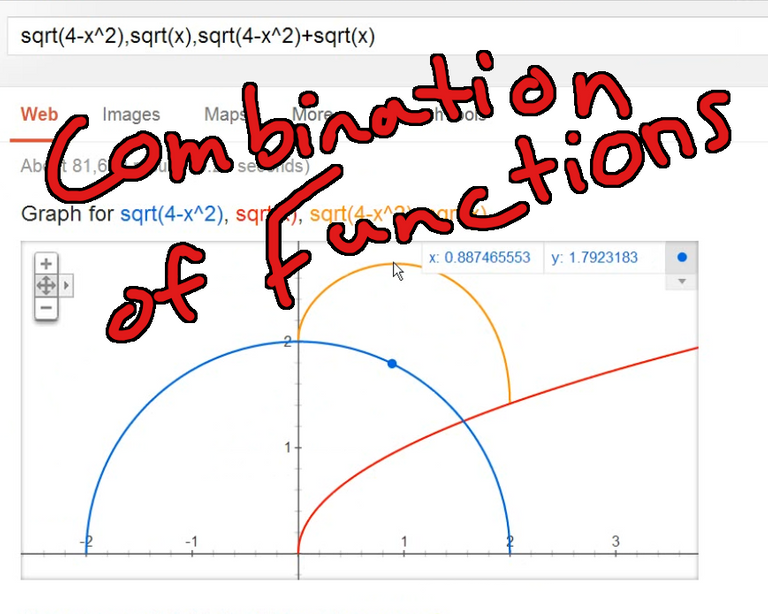# Combination of Functions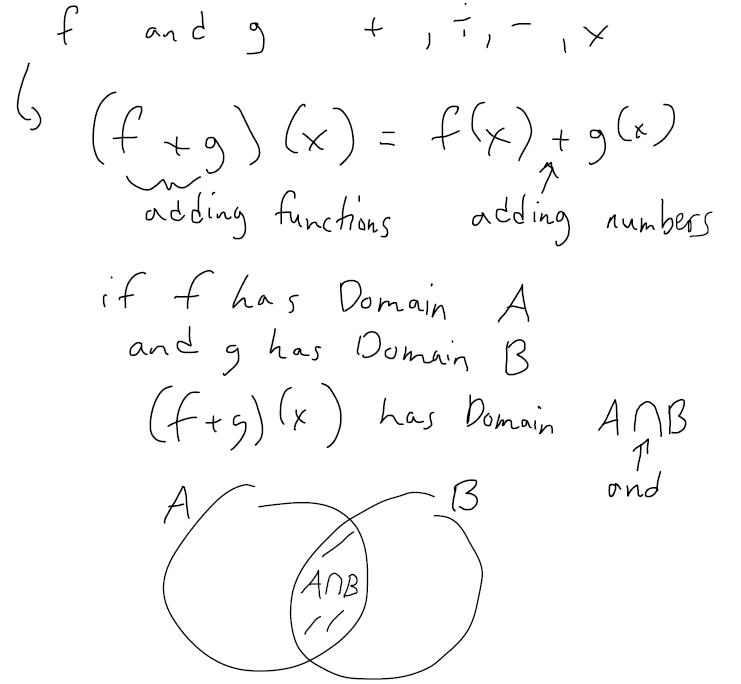## Algebra of Functions

Let f and g be functions with domains A and B.

Then the functions f + g, f – g, fg, and f/g are defined as follows:

• (f + g)(x) = f(x) + g(x) with domain = A ∩ B
• (f – g)(x) = f(x) – g(x) with domain = A ∩ B
• (fg)(x) = f(x)g(x) with domain = A ∩ B
• (f/g)(x) = f(x)/g(x) with domain = {x ϵ A ∩ B|g(x) ≠ 0}
• ϵ = element of or inside.
• | = except
• f/0 is not defined.

## Example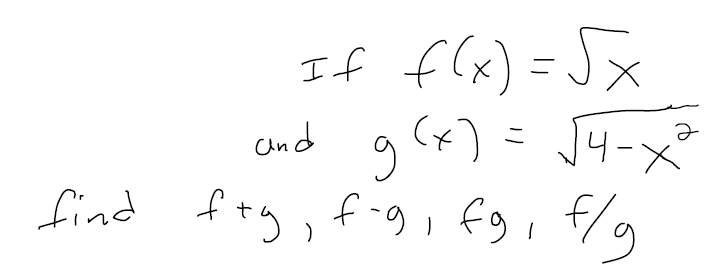### Solution